# List Colourings of Complete Multipartite Graphs with 2 Big Parts

 Importance: Medium ✭✭
 Author(s): Allagan, Julian
 Subject: Graph Theory » Coloring » » Vertex coloring
 Keywords: complete bipartite graph complete multipartite graph list coloring
 Posted by: Jon Noel on: April 12th, 2014
Question   Given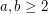, what is the smallest integersuch that?

The list chromatic number of a graph, denoted, is the minimumsuch that for every assignment of lists of sizeto the vertices ofthere is a proper colouring in which every vertex is mapped to a colour in its own list. For more background on the list chromatic number, see .

Given graphsand, the join ofand, denoted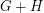, is obtained by taking disjoint copies ofandand adding all edges between them. Ohba  proved that for every graphthere existssuch that. The question above asks to determine the minimum value ofin the case thatis a complete bipartite graph. It seems that it was first studied in , although this is unclear; for the time being, we have chosen to attribute this problem to J. Allagan.

Defineto be the minimumsuch that. Note that, ifis a complete multipartite graph with at most one non-singleton part, then we see that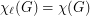by colouring the vertices of the non-singleton part last. Thus, iforis equal to 1, then. As it turns out,and. This can be deduced from the following result of  and the fact that:

Theorem  (Noel, Reed, Wu (2012))   If, then.

The above result of  implies that if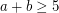, then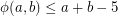. However it seems that, for most values of, this bound is far from tight.

A simple observation is that, since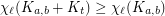for all, we must haveThe following is a result of Allagan :

Theorem  (Allagan (2009))   If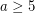, then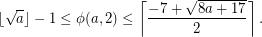This implies thatfor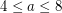and that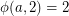for.

## Bibliography

 K. Ohba. On chromatic-choosable graphs, J. Graph Theory. 40 (2002) 130--135. MathSciNet.

 J. A. Noel, B. A. Reed, H. Wu. A Proof of a Conjecture of Ohba. Submitted. pdf.

 J. A. Noel. Choosability of Graphs with Bounded Order: Ohba's Conjecture and Beyond. Master's Thesis, McGill University. pdf.

 J. A. D. Allagan. Choice Numbers, Ohba Numbers and Hall Numbers of some complete-partite graphs. PhD Thesis. Auburn University. 2009.

* indicates original appearance(s) of problem.